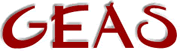[ < ] [ > ] [ << ] [ Up ] [ >> ] [Top] [Contents] [Index] [ ? ]

### 2.3.12 Type conversion

Most user input is in the form of strings; you type something and then the game is supposed to act upon your answer. This calls for functions that can analyze your input and translate it to values you can use in your program. The syntactical analysis is very complicated, to say the least, and I'm going to leave that part for chapter three. However, let's look a bit at the value transformation bit. As stated input is in the form of strings, this makes it very interesting to convert strings to integers and floats, and vice versa.

Let's start with integers. Suppose you have received a string holding a numerical value and you want to use it computations. In order to convert it to the proper integer value you use the efun `atoi()`, very simply. It takes a string as argument and converts it to the corresponding integer. However, if the string contained non-numerical characters apart from leading or trailing spaces, 0 will be returned.

The name `atoi()` is derived from 'a(scii) to i(nteger)', for those of you who are interested to know.

 ```int atoi(string str) e.g. int val; val = atoi("23"); write("23 + 3 = " + (val + 3) + "\n"); ```

Floats have a corresponding efun, `atof()`, which converts a string to float. As you know by now, floats can't be converted to strings the same way integers can by simply adding them to another string, but require some other kind of treatment. The efun `ftoa()` will convert a float to a string, and the reverse functoin `atof()` will turn a string to a float, provided it contains a floating point number. Again, if the string contains any non-numerical characters the result will be 0.

For conversion between integer and float you have the efuns `itof()` and `ftoi()`. Just keep in mind that when you convert a float to integer, the decimal part will be cut off, not rounded.

There are many occasions when you would want to store a value as a string and later convert it back to a value. For this purpose you have the two efuns `val2str()` and `str2val()`. The output from `val2str()` can be printed, but is not intended to. You can store any kind of variable contents as a string using these efuns.

The most used data converter, however, is the efun `sscanf()`. With `sscanf()` you can specify a pattern that should be scanned for a certain value, extract that and put it into a variable. This makes `sscanf()` a bit special since it handles variables given as arguments, so it's impossible to get the function address of `sscanf()`. I know this sounds pretty much like garbled Greek to you at this moment, but trust me. I'll explain more in detail in chapter 3. Anyway, otherwise `sscanf()` is fairly simple; you provide a string to to search through, a pattern and the variables it should store data in, and it returns the number of matches it actually managed to make.

The string you give for pattern matching is interpreted literally apart from these control strings:

`%d'
matches an integer number.

`%s'
matches a character string.

`%f'
matches a float.

`%%'
matches a %-character.

 ```int sscanf(string str, string pattern, ...); e.g. int wide; float weight; string orgstr; string wide_type, weight_type; /* * Assume the question "How wide and heavy do you think Fatty is?" * has been posed and answered to. Furthermore, assume that the * answer is given on the form ' and ', * as for example '4 yards and 3.2 tons'. Assume the first value * always is an integer and that the second is a float. * * Assume that this answer is given in the variable 'orgstr' * * The above is naturally only convenient assumptions to make the * example easy to write. In reality you'd better be prepared for * any kind of format being given as answer. */ if (sscanf(orgstr, "%d %s and %f %s", wide, wide_type, weight, weight_type) != 4) { write("Please give a full answer!\n"); return; } write("Aha, you think Fatty is " + wide + " " + wide_type + " wide and " + ftoa(weight) + " " + weight_type + " heavy.\n"); ```

 [ < ] [ > ] [ << ] [ Up ] [ >> ] [Top] [Contents] [Index] [ ? ]

This document was generated by Ronny Wikh on July, 8 2003 using texi2html